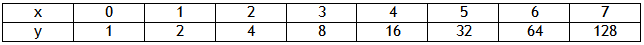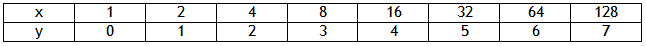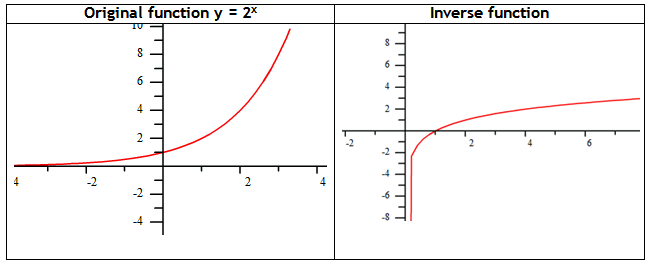Introduction

Just like all families of functions, exponential functions have an inverse. To find the inverse, switch the x and y values of the graph, table or equation to see the new function.

Example Graph the inverse of the function: y = 2x.

Step 1. Make a table of values.Step 2. Switch the values of x and y in the table.Step 3. Plot the points on a coordinate plane.*Note: The asymptote of the original function is y = 0, while the asymptote of the inverse is x = 0. Also, recall from earlier study of inverses, graphs of the inverses are reflected across the line y = x.